# Excel Applications for Accounting Principles

## Quiz 17 :Job Order Costing JobLooking for Accounting Homework Help?

## Quiz 17 :Job Order Costing JobPROBLEM DATA Nutt Products manufactures screws and bolts made to customer specifications. During August, Nutt incurred the following manufacturing costs: direct materials, $28,019.00; direct labor,$15,276.75; and applied factory overhead, $9,854.50. The following data pertain to these costs: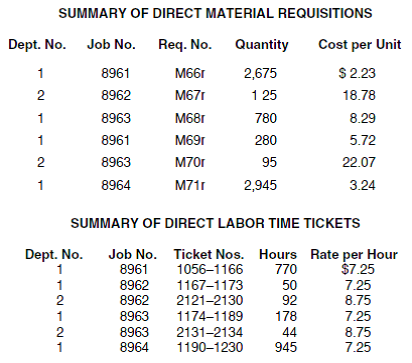The overhead application rates are$4 per direct labor hour for Dept. 1 and 175% of direct labor cost for Dept. 2. Nutt had no beginning work in process for August. Job 8958, which cost $14,190.18 to manufacture, was completed in July and was sold on account in August for$19,000. The job cost sheet for this job is shown on page 103. Of the jobs begun in August, Job 8961 was completed and sold on account for $24,000, Jobs 8962 and 8964 were completed but not sold, and Job 8963 was still in process. REQUIREMENT As cost accountant for this company, you have been asked to prepare job cost sheets for each of the four jobs started in August. Review the printed worksheet called JOB that follows these requirements. Free Essay Answer: Answer: Job order costing is a system of allocating manufacturing costs to a product or an individual or group of products. It is a procedure to appraise the job a company takes up. It helps the company to estimate the costs of materials, labor and other costs to be spent while performing a job. Prepare job cost sheets for each of the four jobs started in August in the following manner: Job 8961: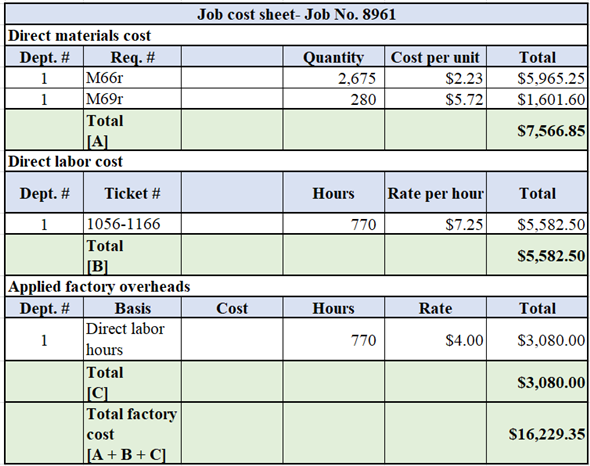Therefore, total factory cost for Job 8961 is$16,229.35.
Job 8962: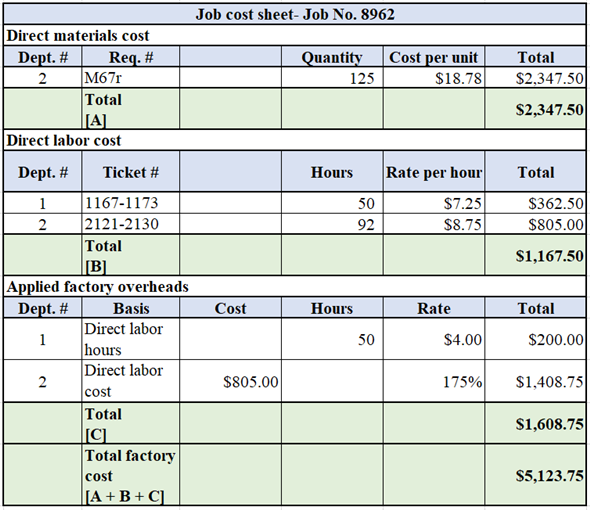Therefore, total factory cost for Job 8962 is $5,123.75. Job 8963: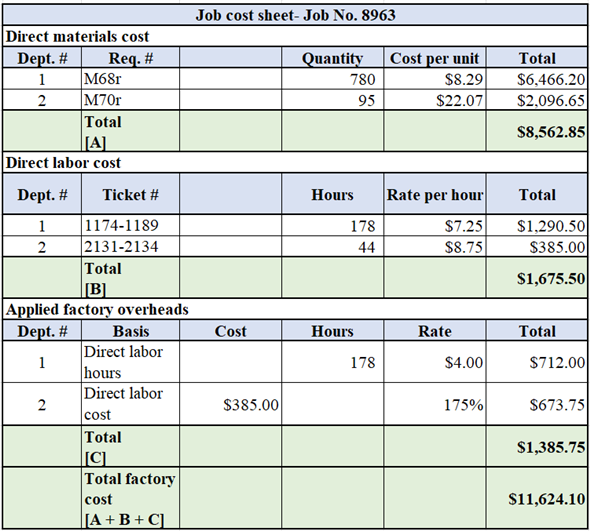Therefore, total factory cost for Job 8963 is$11,624.10.
Job 8964 :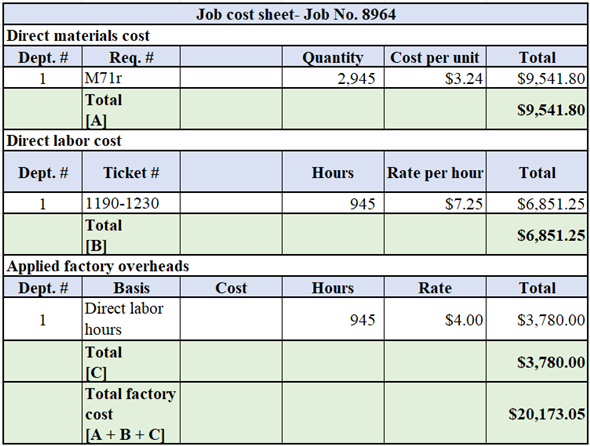Therefore, total factory cost for Job 8964 is $20,173.05.PROBLEM DATA Nutt Products manufactures screws and bolts made to customer specifications. During August, Nutt incurred the following manufacturing costs: direct materials,$28,019.00; direct labor, $15,276.75; and applied factory overhead,$9,854.50. The following data pertain to these costs:The overhead application rates are $4 per direct labor hour for Dept. 1 and 175% of direct labor cost for Dept. 2. Nutt had no beginning work in process for August. Job 8958, which cost$14,190.18 to manufacture, was completed in July and was sold on account in August for $19,000. The job cost sheet for this job is shown on page 103. Of the jobs begun in August, Job 8961 was completed and sold on account for$24,000, Jobs 8962 and 8964 were completed but not sold, and Job 8963 was still in process. REQUIREMENT Open the file JOB from the website for this book at cengagebrain.com. There are 13 fairly simple formulas to enter on this worksheet. Using the printout of Job 8958, you can determine that FORMULA1 must be =E12*F12. Enter the 13 formulas in the appropriate cells on the worksheet. This worksheet will be used to compute the total cost of each job worked on. It will simplify matters to save the completed version of JOB before entering specific job data on it. Save your empty worksheet with the formulas on it as JOB2. Also print your formulas.
Free
Essay

Enter the formulas where indicated in the job cost sheet where indicated using cell references. In the direct materials section, the formulas will be quantity multiplied by cost per unit. In the direct labor section, the formulas will be hours multiplied by rate. The ending totals will be calculated by adding the numbers for that section. Because there is no data entered yet, formulas will give the result of $0.00. Formula 8 will be the total number of hours of direct labor and Formula 9 will be the total direct materials cost. Worksheet showing job cost sheet: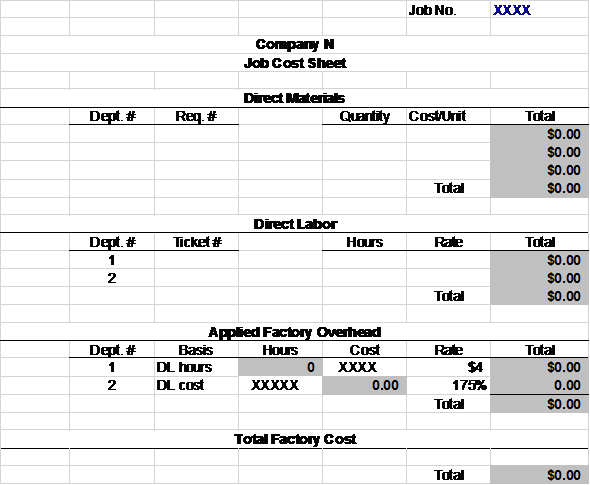WHAT-IF ANALYSIS Now that your worksheet has been saved as JOB2, fill in the appropriate data for Job 8961. Save the results as JOB8961 and print the completed job cost sheet when done. Check figure: Cost of Job 8961 (cell G31),$16,229.35.
Free
Essay

Using given data, enter the information for Job 8961 into the appropriate sections of the job cost sheet. Worksheet showing job cost sheet: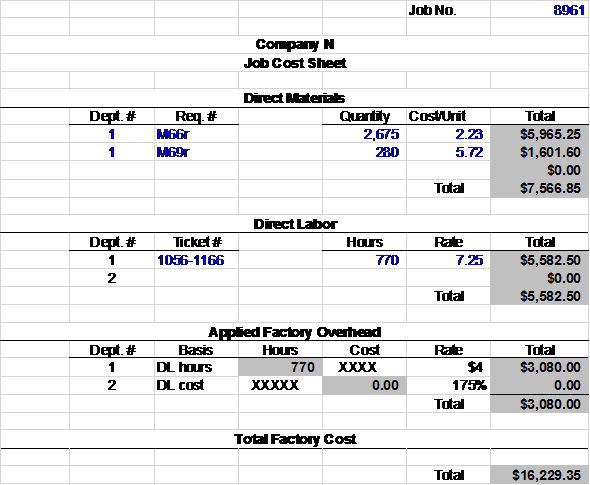WHAT-IF ANALYSIS Next, open worksheet JOB2 again. Enter the data for Job 8962, save the results as JOB8962, and print the worksheet. Repeat these steps for Jobs 8963 and 8964.
EssayCHART ANALYSIS Nutt's management is very concerned about the cost of overhead on its jobs. When jobs are complete, overhead costs should be between 15% and 20% of total costs. For example, the labor cost on Job 8958 is 25% of total costs, higher than the norm. Open Job 8961 and click the Chart sheet tab. A pie chart appears showing the cost components on that job. Record the labor cost percentage in the space provided. Repeat this for each of the jobs worked on in August.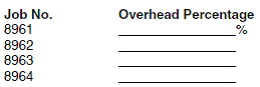Did Nutt maintain good cost control on all its jobs Explain.